# Graph of a function of two variables

## Definition

Suppose$f$ is a function of two variables$x,y$, with domain a subset$S$ of$\R^2$. The graph of$f$ is a subset of three-dimensional Euclidean space$\R^3$ with coordinates$x,y,z$, given by the equation:$\! z = f(x,y)$

Equivalently, it is the set of points:$\{ (x,y,f(x,y)) \mid (x,y) \in S \}$

Pictorially, this graph looks like a surface for a nice enough function$f$.

Another way of defining the graph is that for every point$(x_0,y_0) \in S$, there is precisely one point of the graph on the line$x = x_0, y = y_0$, namely the point with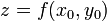$z = f(x_0,y_0)$.

The$x$- and$y$-axes are the independent variable axes and the$z$-axis, also called the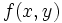$f(x,y)$-axis, is the dependent variable axis.

## Aspects

### Domain and range

Aspect of the function How it can be deduced from the graph
domain project the entire graph on the$xy$-plane.
range project the entire graph on the$z$-axis.

### Vertical line test

The vertical line test for a function of one variable says that every vertical line intersects the graph in exactly one point if the$x$-coordinate is in the domain and in no point if the$x$-coordinate is not in the domain. There is an analogous test for a function of two variables. This says that any line parallel to the$z$-axis (the function value axis) intersects the graph in exactly one point if the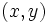$(x,y)$-pair for the line is in the domain, and intersects the graph in no point if the$(x,y)$-pair for the line is not in the domain. In particular, any line parallel to the$z$-axis must intersect the graph of a function in at most one point.

If a particular subset of$\R^3$ violates this condition, it cannot be realized as the graph of a function.

## Restriction to one variable

### Graph of the restriction

Suppose we fix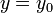$y = y_0$ but allow$x$ to vary. On the domain side, this is equivalent to considering the horizontal line$y = y_0$ in the$xy$-plane. Suppose we are interested in studying the restriction of the function to this line (or more precisely, the intersection of this line with$S$). In other words, we are interested in studying the function: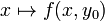$x \mapsto f(x,y_0)$

This is a function of one variable, namely$x$. Further, the graph of this function can be obtained by intersecting the graph of the original function with the plane$y = y_0$ in$\R^3$. Note that this plane is parallel to the$xz$-plane. In this plane, we treat$x$ as the independent variable and$z$ as the dependent variable.

Analogously, suppose we fix$x = x_0$ but allow$y$ to vary. On the domain side, this is equivalent to considering the vertical line$x = x_0$ in the$xy$-plane. Suppose we are interested in studying the restriction of the function to this line (or more precisely, the intersection of this line with$S$). In other words, we are interested in studying the function: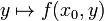$y \mapsto f(x_0,y)$

This is a function of one variable, namely$y$. Further, the graph of this function can be obtained by intersecting the graph of the original function with the plane$x = x_0$ in$\R^3$. Note that this plane is parallel to the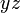$yz$-plane. In this plane, we treat$y$ as the independent variable and$z$ as the dependent variable.

### Continuity in each variable and separate continuity in graphical terms

We have the following:

Assertion about continuity How we can verify it from the graph$f$ is continuous in$x$ at the point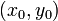$(x_0,y_0)$ Consider the graph restricted to the plane$y= y_0$. This is continuous at$x = x_0$.$f$ is continuous in$y$ at the point$(x_0,y_0)$ Consider the graph restricted to the plane$x= x_0$. This is continuous at$y = y_0$.$f$ is separately continuous continuous in both variables at the point$(x_0,y_0)$. Both the above conditions.$f$ is continuous in$x$ everywhere. The restrictions of the graph to all planes parallel to the$xz$-plane give graphs of continuous functions.$f$ is continuous in$y$ everywhere. The restrictions of the graph to all planes parallel to the$yz$-plane give graphs of continuous functions.$f$ is separately continuous in both variables everywhere. Both the above conditions, i.e., the restrictions of the graph to all planes parallel to either the$xz$-plane or the$yz$-plane are graphs of continuous functions.

### Partial derivatives in graphical terms

For further information, refer: partial derivative

We have the following:

Partial derivative Graphical interpretation
The partial derivative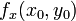$f_x(x_0,y_0)$ at a point$(x_0,y_0)$ in the domain of the function The slope of the tangent line at$x= x_0$ to the restriction of the graph of$f$ to the plane$y = y_0$.
The partial derivative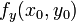$f_y(x_0,y_0)$ at a point$(x_0,y_0)$ in the domain of the function The slope of the tangent line at$y = y_0$ to the restriction of the graph of$f$ to the plane$x = x_0$.

### Directional derivatives in graphical terms

For further information, refer: directional derivative

The directional derivative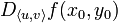$D_{\langle u,v \rangle} f(x_0,y_0)$ in the direction of a unit vector$\langle u,v \rangle$ at a point$(x_0,y_0)$ can be determined as follows: first, intersect the graph of the function with the plane$v(x - x_0) = u(y - y_0)$. This plane is perpendicular to the$xy$-plane and its intersection with the$xy$-plane is the line through$(x_0,y_0)$ in the direction of the unit vector$\langle u,v \rangle$.

This intersection can be thought of as the graph of a function of one variable, where the point$(x_0,y_0,0)$ is treated as the origin, the direction$(u,v,0)$ is the independent variable axis, and the$z$-axis direction is the dependent variable axis. Now, the directional derivative is the slope of this graph for dependent variable value of 0.

### Gradient vector in graphical terms

For further information, refer: gradient vector

We say that$f$ is differentiable at a point$(x_0,y_0)$ if the gradient vector exists at the point. This is equivalent to the graph of the function having a well defined tangent plane at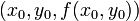$(x_0,y_0,f(x_0,y_0))$. Further, the equation of this tangent plane is given by: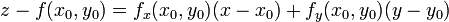$z - f(x_0,y_0) = f_x(x_0,y_0)(x - x_0) + f_y(x_0,y_0)(y - y_0)$

Another way of putting this is:$z - f(x_0,y_0) = (\nabla f)(x_0,y_0) \cdot (\langle x,y \rangle - \langle x_0,y_0\rangle)$

Note that it is possible that the partial derivatives both exist but the function is not differentiable. In this case, the surface does not have a well defined tangent plane at the point. Even though we can define a plane by the equation above, this is not the tangent plane, because the tangent plane does not exist.